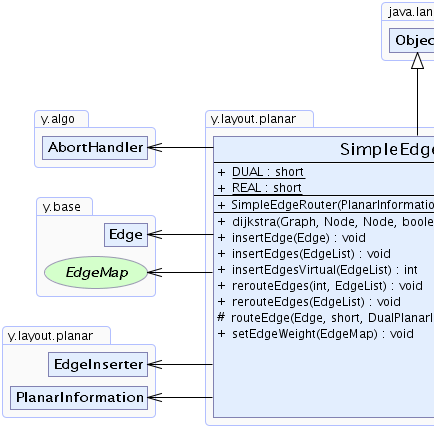Search this API

## y.layout.planar Class SimpleEdgeRouter

```java.lang.Objecty.layout.planar.SimpleEdgeRouter
```

`public class SimpleEdgeRouterextends java.lang.Object`

Instances of this class route edges through a planar graph using its dual graph.Field Summary
`static short` `DUAL`

`static short` `REAL`

Constructor Summary
```SimpleEdgeRouter(PlanarInformation _planar, long maximumDuration)```

Method Summary
` void` ```dijkstra(Graph graph, Node s, Node t, boolean directed, int[] cost, int[] dist, Edge[] pred)```

` void` `insertEdge(Edge currentEdge)`
Inserts a single edge into the graph by routing through the dual graph.
` void` `insertEdges(EdgeList edges)`
Inserts a list of edges into the graph by routing through the dual graph.
` int` `insertEdgesVirtual(EdgeList edges)`

` void` `rerouteEdges(EdgeList edges)`
Tries to find better paths for all edges that caused crossings.
` void` ```rerouteEdges(int iter, EdgeList edges)```
Tries to find better paths for all edges that caused crossings.
`protected  EdgeList` ```routeEdge(Edge e, short _pathStyle, DualPlanarInformation dualPlanar, Edge[] zeroEdges)```
This method tries to find the best route through a graph.
` void` `setEdgeWeight(EdgeMap edgeWeight)`

Methods inherited from class java.lang.Object
`clone, equals, finalize, getClass, hashCode, notify, notifyAll, toString, wait, wait, wait`

Field Detail

### DUAL

`public static final short DUAL`
Constant Field Values

### REAL

`public static final short REAL`
Constant Field Values
Constructor Detail

### SimpleEdgeRouter

```public SimpleEdgeRouter(PlanarInformation _planar,
long maximumDuration)```
Method Detail

### setEdgeWeight

`public void setEdgeWeight(EdgeMap edgeWeight)`

### insertEdges

`public void insertEdges(EdgeList edges)`
Inserts a list of edges into the graph by routing through the dual graph.

Parameters:
`edges` - the edges to be routed routed.

### insertEdgesVirtual

`public int insertEdgesVirtual(EdgeList edges)`

### insertEdge

`public void insertEdge(Edge currentEdge)`
Inserts a single edge into the graph by routing through the dual graph.

Parameters:
`currentEdge` - the edge to be routed.

### routeEdge

```protected EdgeList routeEdge(Edge e,
short _pathStyle,
DualPlanarInformation dualPlanar,
Edge[] zeroEdges)```
This method tries to find the best route through a graph. Best means that the route causes as less crossings as possible.

Precondition:
a valid dual graph, dsp must be initialized
Parameters:
`e` - the edge to be routed
Returns:
the path through the dual graph that yielded the best result

### rerouteEdges

```public void rerouteEdges(int iter,
EdgeList edges)```
Tries to find better paths for all edges that caused crossings.

Precondition:
is a valid dual graph
Parameters:
`iter` - number of iterations to perform. A value less or equal to zero causes the method to run until the best possible result is found.

### rerouteEdges

`public void rerouteEdges(EdgeList edges)`
Tries to find better paths for all edges that caused crossings.

### dijkstra

```public void dijkstra(Graph graph,
Node s,
Node t,
boolean directed,
int[] cost,
int[] dist,
Edge[] pred)```